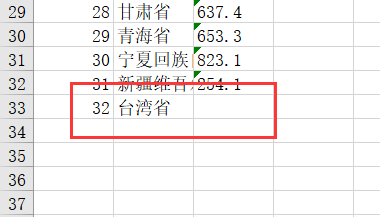TheGkeone 阅读(18) 评论(0)

【最近一些朋友反映个别代码无法调试（但是在我这里没问题），这里代码我都是调试过的，之前一年也没有朋友反馈过这些问题，所以大概率是版本问题。

1、使用xlrd模块对xls文件进行读操作

1.1 获取工作簿对象

1.2 获取工作表对象

1.3 获取工作表的基本信息

1.4 按行或列方式获得工作表的数据

1.5 获取某一个单元格的数据

2、使用xlwt模块对xls文件进行写操作

2.1 创建工作簿

2.2 创建工作表

2.3 按单元格的方式向工作表中添加数据

2.4 按行或列方式向工作表中添加数据

2.5 保存创建的文件

3、使用openpyxl模块对xlsx文件进行读操作

3.1 获取工作簿对象

3.2 获取所有工作表名

3.3 获取工作表对象

3.4 根据索引方式获取工作表对象

3.5 获取工作表的属性

3.6 按行或列方式获取表中的数据

3.7 获取特定行或特定列的数据

3.8 获取某一块的数据

3.9 获取某一单元格的数据

4、使用openpyxl模块对xlsx文件进行写操作

4.1 创建工作簿和获取工作表

4.2 创建新的工作表

4.3 将数据写入工作表

4.4 保存工作簿

5、修改已经存在的工作簿(表)

5.1 插入一列数据

5.2 修改特定单元格

5.3 批量修改数据

# 1、使用xlrd模块对xls文件进行读操作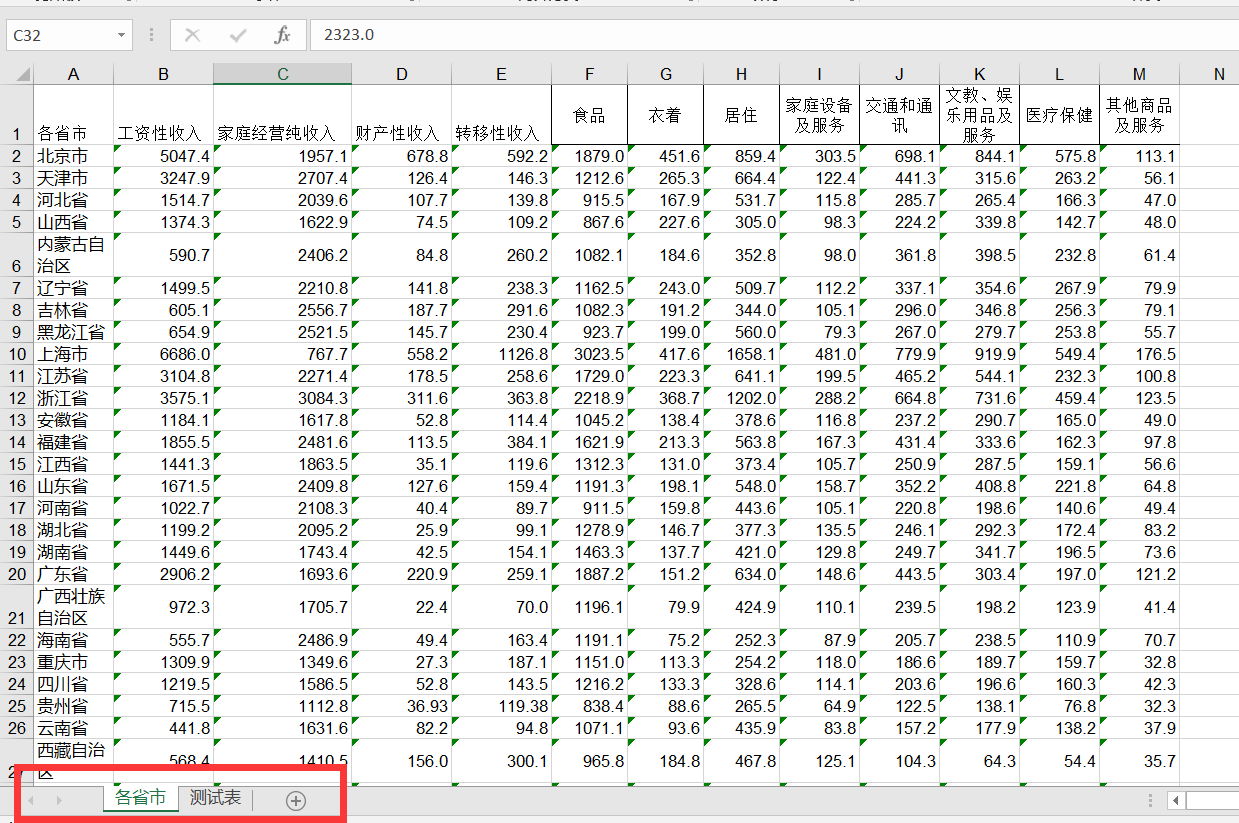## 1.1 获取工作簿对象

``````
import xlrd  #引入模块

#打开文件，获取excel文件的workbook（工作簿）对象
workbook=xlrd.open_workbook("DataSource/Economics.xls")  #文件路径

``````

## 1.2 获取工作表对象

``````'''对workbook对象进行操作'''

#获取所有sheet的名字
names=workbook.sheet_names()
print(names) #['各省市', '测试表']  输出所有的表名，以列表的形式

#通过sheet索引获得sheet对象
worksheet=workbook.sheet_by_index(0)
print(worksheet)  #<xlrd.sheet.Sheet object at 0x000001B98D99CFD0>

#通过sheet名获得sheet对象
worksheet=workbook.sheet_by_name("各省市")
print(worksheet) #<xlrd.sheet.Sheet object at 0x000001B98D99CFD0>

#由上可知，workbook.sheet_names() 返回一个list对象，可以对这个list对象进行操作
sheet0_name=workbook.sheet_names()  #通过sheet索引获取sheet名称
print(sheet0_name)  #各省市

``````

## 1.3 获取工作表的基本信息

``````'''对sheet对象进行操作'''
name=worksheet.name  #获取表的姓名
print(name) #各省市

nrows=worksheet.nrows  #获取该表总行数
print(nrows)  #32

ncols=worksheet.ncols  #获取该表总列数
print(ncols) #13
``````

## 1.4 按行或列方式获得工作表的数据

``````for i in range(nrows): #循环打印每一行
print(worksheet.row_values(i))  #以列表形式读出，列表中的每一项是str类型
#['各省市', '工资性收入', '家庭经营纯收入', '财产性收入', ………………]
#['北京市', '5047.4', '1957.1', '678.8', '592.2', '1879.0，…………]

col_data=worksheet.col_values(0)  #获取第一列的内容
print(col_data)

``````

## 1.5 获取某一个单元格的数据

我们还可以将查询精确地定位到某一个单元格。

``````#通过坐标读取表格中的数据
cell_value1=sheet0.cell_value(0,0)
cell_value2=sheet0.cell_value(1,0)
print(cell_value1)  #各省市
print(cell_value2)   #北京市

cell_value1=sheet0.cell(0,0).value
print(cell_value1) #各省市
cell_value1=sheet0.row(0).value
print(cell_value1)  #各省市``````

# 2、使用xlwt模块对xls文件进行写操作

## 2.1 创建工作簿

``````
# 导入xlwt模块
import xlwt

#创建一个Workbook对象，相当于创建了一个Excel文件
book=xlwt.Workbook(encoding="utf-8",style_compression=0)

'''
Workbook类初始化时有encoding和style_compression参数
encoding:设置字符编码，一般要这样设置：w = Workbook(encoding='utf-8')，就可以在excel中输出中文了。默认是ascii。
style_compression:表示是否压缩，不常用。
'''
``````

## 2.2 创建工作表

``````
# 创建一个sheet对象，一个sheet对象对应Excel文件中的一张表格。
sheet = book.add_sheet('test01', cell_overwrite_ok=True)
# 其中的test是这张表的名字,cell_overwrite_ok，表示是否可以覆盖单元格，其实是Worksheet实例化的一个参数，默认值是False
``````

## 2.3 按单元格的方式向工作表中添加数据

``````
# 向表test中添加数据
sheet.write(0, 0, '各省市')  # 其中的'0-行, 0-列'指定表中的单元，'各省市'是向该单元写入的内容
sheet.write(0, 1, '工资性收入')

#也可以这样添加数据
txt1 = '北京市'
sheet.write(1,0, txt1)
txt2 = 5047.4
sheet.write(1, 1, txt2)``````

最后被文件被保存之后，上文语句形成的“工作表”如下所示: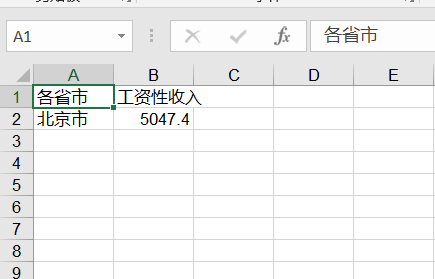## 2.4 按行或列方式向工作表中添加数据

``````
#添加第二个表
sheet2=book.add_sheet("test02",cell_overwrite_ok=True)

Province=['北京市', '天津市', '河北省', '山西省', '内蒙古自治区', '辽宁省',
'吉林省', '黑龙江省', '上海市', '江苏省', '浙江省', '安徽省', '福建省',
'江西省', '山东省', '河南省', '湖北省', '湖南省', '广东省', '广西壮族自治区',
'海南省', '重庆市', '四川省', '贵州省', '云南省', '西藏自治区', '陕西省', '甘肃省',
'青海省', '宁夏回族自治区', '新疆维吾尔自治区']

Income=['5047.4', '3247.9', '1514.7', '1374.3', '590.7', '1499.5', '605.1', '654.9',
'6686.0', '3104.8', '3575.1', '1184.1', '1855.5', '1441.3', '1671.5', '1022.7',
'1199.2', '1449.6', '2906.2', '972.3', '555.7', '1309.9', '1219.5', '715.5', '441.8',
'568.4', '848.3', '637.4', '653.3', '823.1', '254.1']

Project=['各省市', '工资性收入', '家庭经营纯收入', '财产性收入', '转移性收入', '食品', '衣着',
'居住', '家庭设备及服务', '交通和通讯', '文教、娱乐用品及服务', '医疗保健', '其他商品及服务']

#填入第一列
for i in range(0, len(Province)):
sheet2.write(i+1, 0, Province[i])

#填入第二列
for i in range(0,len(Income)):
sheet2.write(i+1,1,Income[i])

#填入第一行
for i in range(0,len(Project)):
sheet2.write(0,i,Project[i])``````

## 2.5 保存创建的文件

``````# 最后，将以上操作保存到指定的Excel文件中
book.save('DataSource\\test1.xls')  ``````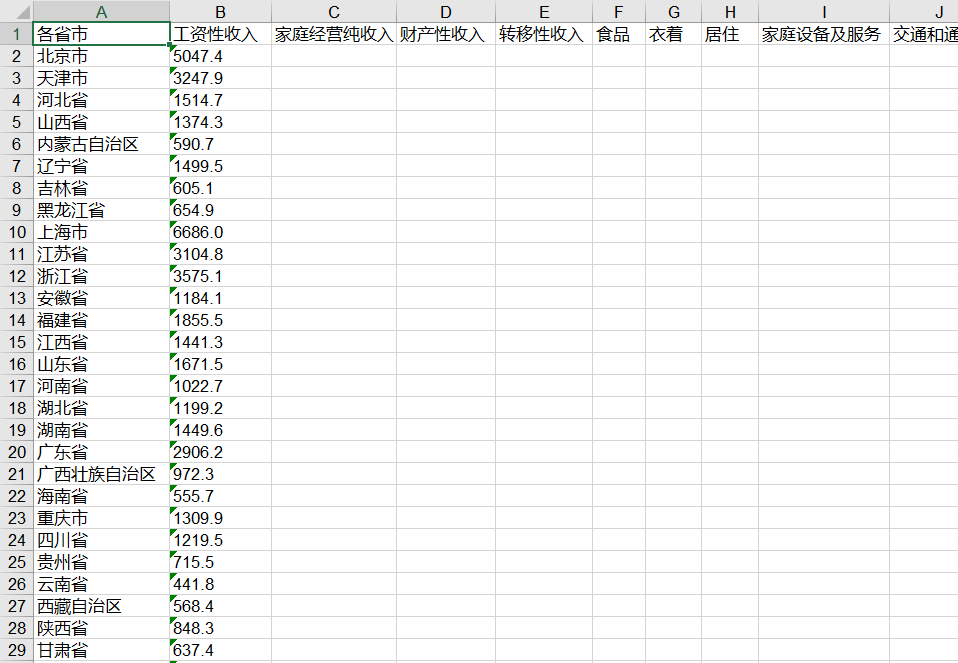# 3、使用openpyxl模块对xlsx文件进行读操作

## 3.1 获取工作簿对象

``````import openpyxl

#获取 工作簿对象
workbook=openpyxl.load_workbook("DataSource\Economics.xlsx")
#与xlrd 模块的区别
#wokrbook=xlrd.open_workbook(""DataSource\Economics.xls)``````

## 3.2 获取所有工作表名

``````#获取工作簿 workbook的所有工作表
shenames=workbook.get_sheet_names()
print(shenames)  #['各省市', '测试表']
#在xlrd模块中为 sheetnames=workbook.sheet_names()

#使用上述语句会发出警告：DeprecationWarning: Call to deprecated function get_sheet_names (Use wb.sheetnames).
#说明 get_sheet_names已经被弃用 可以改用 wb.sheetnames 方法
shenames=workbook.sheetnames
print(shenames)  #['各省市', '测试表']
``````

## 3.3 获取工作表对象

``````#获得工作簿的表名后，就可以获得表对象
worksheet=workbook.get_sheet_by_name("各省市")
print(worksheet)  #<Worksheet "各省市">

#使用上述语句同样弹出警告：DeprecationWarning: Call to deprecated function get_sheet_by_name (Use wb[sheetname]).
#改写成如下格式
worksheet=workbook["各省市"]
print(worksheet)  #<Worksheet "各省市">

#还可以通过如下写法获得表对象
worksheet1=workbook[shenames]
print(worksheet1)  #<Worksheet "测试表">``````

## 3.4 根据索引方式获取工作表对象

``````#还可以通过索引方式获取表对象
worksheet=workbook.worksheets
print(worksheet) #<Worksheet "各省市">

#也可以用如下方式
#获取当前活跃的worksheet,默认就是第一个worksheet
ws = workbook.active``````

## 3.5 获取工作表的属性

``````#经过上述操作，我们已经获得了第一个“表”的“表对象“，接下来可以对表对象进行操作

name=worksheet.title  #获取表名
print(name)  #各省市
#在xlrd中为worksheet.name

#获取该表相应的行数和列数
rows=worksheet.max_row
columns=worksheet.max_column
print(rows,columns)  #32 13
#在xlrd中为 worksheet.nrows  worksheet.ncols``````

## 3.6 按行或列方式获取表中的数据

sheet.rows，这是一个生成器，里面是每一行数据，每一行数据由一个元组类型包裹。

sheet.columns，同上，里面是每一列数据。

``````for row in worksheet.rows:
for cell in row:
print(cell.value,end=" ")
print()
"""

……
"""

for col in worksheet.columns:
for cell in col:
print(cell.value,end=" ")
print()

'''

……
'''``````

``````
for row in worksheet.rows:
print(row)
'''
(<Cell '各省市'.A1>, <Cell '各省市'.B1>, <Cell '各省市'.C1>, <Cell '各省市'.D1>, <Cell '各省市'.E1>,……
(<Cell '各省市'.A2>, <Cell '各省市'.B2>, <Cell '各省市'.C2>, <Cell '各省市'.D2>, <Cell '各省市'.E2>, ……
……
'''
#可知，需要二次迭代

for row in worksheet.rows:
for cell in row:
print(cell,end=" ")
print()

'''
<Cell '各省市'.A1> <Cell '各省市'.B1> <Cell '各省市'.C1> <Cell '各省市'.D1>……
<Cell '各省市'.A2> <Cell '各省市'.B2> <Cell '各省市'.C2> <Cell '各省市'.D2> ……
……
'''
#还需要cell.value
for row in worksheet.rows:
for cell in row:
print(cell.value,end=" ")
print()
``````

## 3.7 获取特定行或特定列的数据

``````#输出特定的行
for cell in list(worksheet.rows):  #获取第四行的数据
print(cell.value,end=" ")
print()
#河北省 1514.7 2039.6 107.7 139.8 915.5 167.9 531.7 115.8 285.7 265.4 166.3 47.0

#输出特定的列
for cell in list(worksheet.columns):  #获取第三列的数据
print(cell.value,end=" ")
print()
#家庭经营纯收入 1957.1 2707.4 2039.6 1622.9 2406.2 2210.8 2556.7 2521.5 767.7 2271.4 3084.3……

#已经转换成list类型，自然是从0开始计数。``````

## 3.8 获取某一块的数据

``````for rows in list(worksheet.rows)[0:3]:
for cell in rows[0:3]:
print(cell.value,end=" ")
print()
'''

'''

for i in range(1, 4):
for j in range(1, 4):
print(worksheet.cell(row=i, column=j).value,end=" ")
print()
'''

'''``````

## 3.9 获取某一单元格的数据

``````#精确读取表格中的某一单元格
content_A1= worksheet['A1'].value
print(content_A1)

content_A1=worksheet.cell(row=1,column=1).value
#等同于 content_A1=worksheet.cell(1,1).value
print(content_A1)
#此处的行数和列数都是从1开始计数的，而在xlrd中是由0开始计数的``````

# 4、使用openpyxl模块对xlsx文件进行写操作

## 4.1 创建工作簿和获取工作表

``````import openpyxl

# 创建一个Workbook对象，相当于创建了一个Excel文件
workbook=openpyxl.Workbook()
#wb=openpyxl.Workbook(encoding='UTF-8')

#获取当前活跃的worksheet,默认就是第一个worksheet
worksheet = workbook.active
worksheet.title="mysheet"``````

## 4.2 创建新的工作表

``````worksheet2 = workbook.create_sheet()   #默认插在工作簿末尾
#worksheet2 = workbook.create_sheet(0)  #插入在工作簿的第一个位置
worksheet2.title = "New Title"``````

## 4.3 将数据写入工作表

``````#以下是我们要写入的数据
Province=['北京市', '天津市', '河北省', '山西省', '内蒙古自治区', '辽宁省',
'吉林省', '黑龙江省', '上海市', '江苏省', '浙江省', '安徽省', '福建省',
'江西省', '山东省', '河南省', '湖北省', '湖南省', '广东省', '广西壮族自治区',
'海南省', '重庆市', '四川省', '贵州省', '云南省', '西藏自治区', '陕西省', '甘肃省',
'青海省', '宁夏回族自治区', '新疆维吾尔自治区']

Income=['5047.4', '3247.9', '1514.7', '1374.3', '590.7', '1499.5', '605.1', '654.9',
'6686.0', '3104.8', '3575.1', '1184.1', '1855.5', '1441.3', '1671.5', '1022.7',
'1199.2', '1449.6', '2906.2', '972.3', '555.7', '1309.9', '1219.5', '715.5', '441.8',
'568.4', '848.3', '637.4', '653.3', '823.1', '254.1']

Project=['各省市', '工资性收入', '家庭经营纯收入', '财产性收入', '转移性收入', '食品', '衣着',
'居住', '家庭设备及服务', '交通和通讯', '文教、娱乐用品及服务', '医疗保健', '其他商品及服务']

#写入第一行数据，行号和列号都从1开始计数
for i in range(len(Project)):
worksheet.cell(1, i+1,Project[i])

#写入第一列数据，因为第一行已经有数据了，i+2
for i in range(len(Province)):
worksheet.cell(i+2,1,Province[i])

#写入第二列数据
for i in range(len(Income)):
worksheet.cell(i+2,2,Income[i])
``````

## 4.4 保存工作簿

``workbook.save(filename='DataSource\\myfile.xlsx')``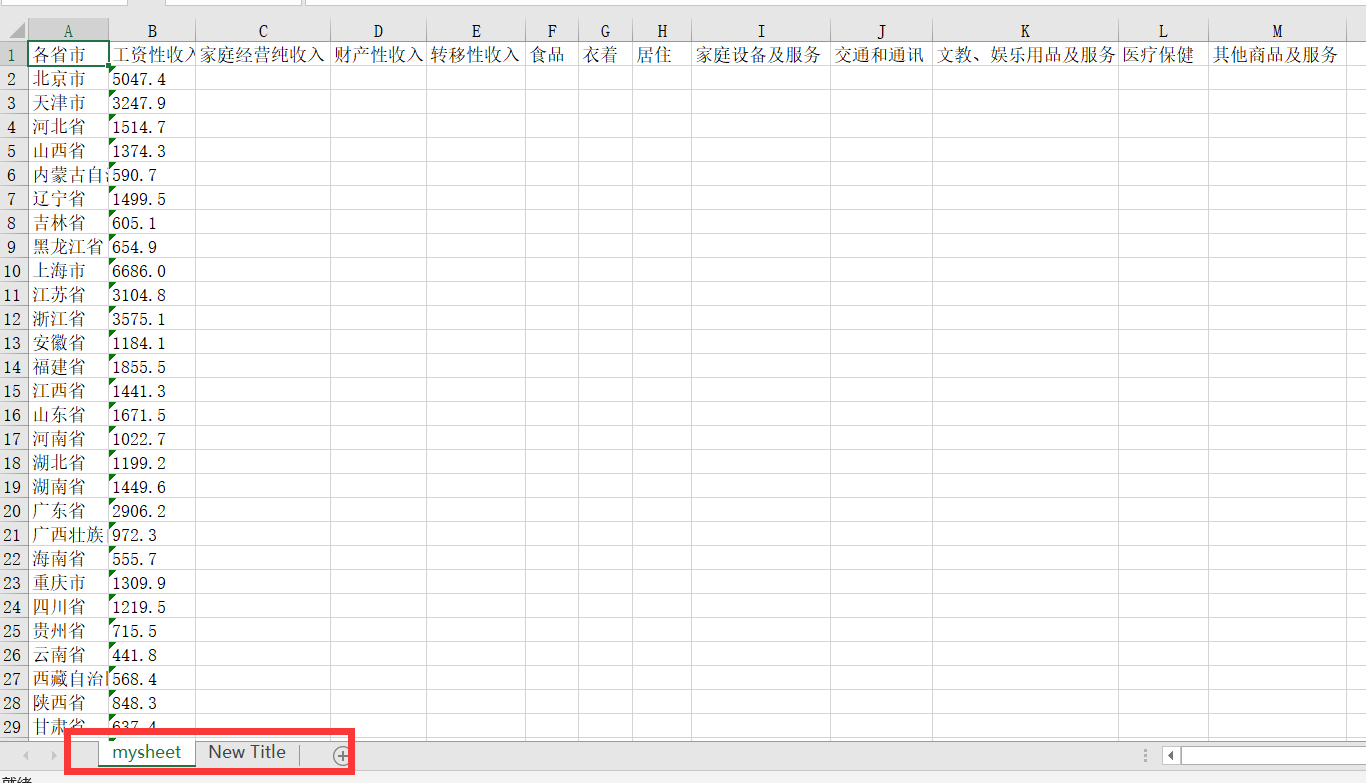# 5、修改已经存在的工作簿(表)

## 5.1 插入一列数据

``````import openpyxl

workbook=openpyxl.load_workbook("DataSource\myfile.xlsx")
worksheet=workbook.worksheets

#在第一列之前插入一列
worksheet.insert_cols(1)  #

for index,row in enumerate(worksheet.rows):
if index==0:
row.value="编号"  #每一行的一个row就是第一列
else:
row.value=index
#枚举出来是tuple类型，从0开始计数

workbook.save(filename="DataSource\myfile.xlsx")``````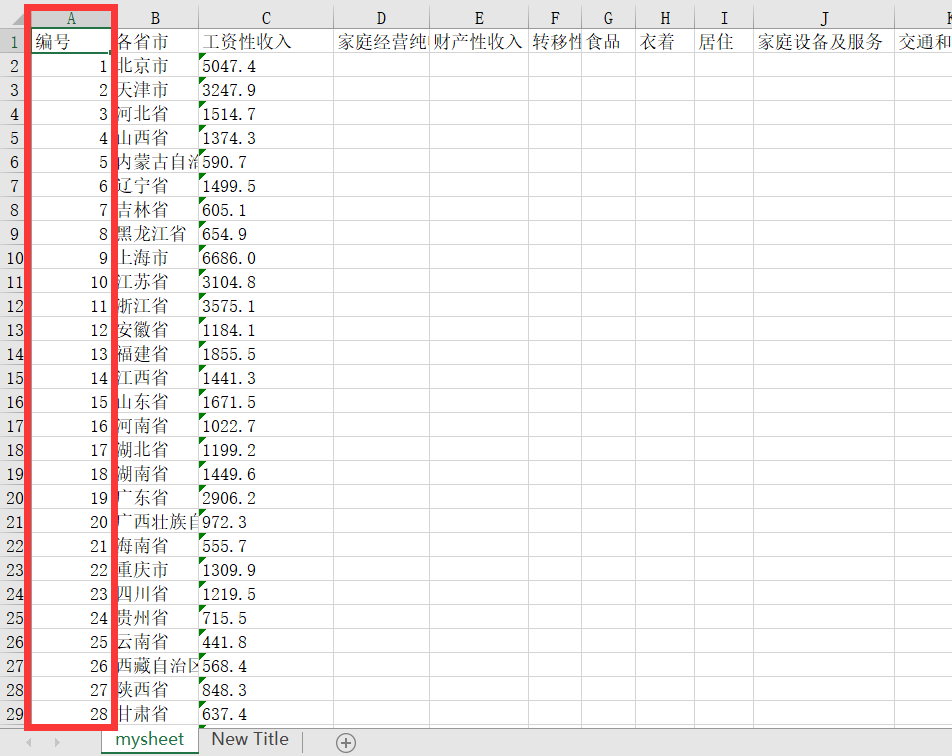## 5.2 修改特定单元格

``````worksheet.cell(2,3,'0')
worksheet["B2"]="Peking"``````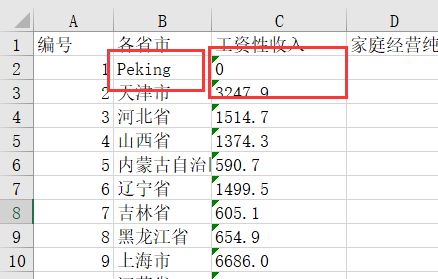## 5.3 批量修改数据

``````taiwan=[32,"台湾省"]
worksheet.append(taiwan)``````